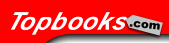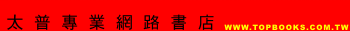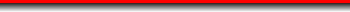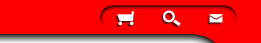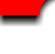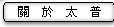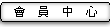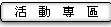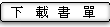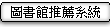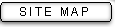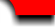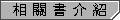[數理] Introduction to Probability: Models and Applications ( 2020 ) [數理] Statistical Analysis of Financial Data: With Examples in R ( 2020 ) [數理] Probability: Theory, Examples, Problems, Simulations ( 2020 ) [數理] Probability and Mathematical Statistics: Theory, Applications, and Practice in R ( 2019 ) [數理] Controlled Branching Processes ( 2018 ) [數理] Probability and Random Number: A First Guide to Randomness ( 2018 ) [數理] Statistical Data Fusion ( 2017 ) [數理] Statistics: Second Edition of ’A Second Course in Statistics’ ( 2016 ) [數理] Mortality and Other Investigations, vol. 1 ( 2015 ) [數理] Change of Time and Change of Measure ( 2010 )ISBN10：1642952834
ISBN13：9781642952834

English / 平裝
[ 數理 ] 統計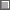觀看書評總覽好書推薦親友發表推薦感言

....................................................................................................................